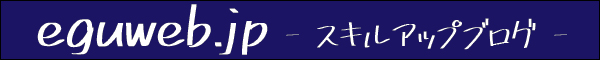# Google Spreadsheet How to calculate total operating hours (working hours) from the displayed time (hh:mm)

This method calculates work hours from the displayed time (hh:mm).

## Time clocked out – Time clocked in

So, let’s subtract the time of leaving work – time of coming to work.

I got a result that looks like that.

Is that it?

Wait a minute…!

Let’s continue.

## What is the total operating hours (working hours)…?

If we add up the working hours as is, the total working hours will look strange.

Let’s sum the columns using SUM.

Let’s sum up.

I don’t understand the result…

## Calculate by integer value (in hours) (time*24,0)

The formula is 1:00 = 1/(24*60), so we can apply it here to find it.

In other words, if you want to calculate in hours, multiply by 24 to obtain.

Total operating hours = sum(X:X)*24

We get a result of 39.23 hours worked (operating hours)…!

## Calculate with integer value (in minutes) (time*1440,0)

If you want to get the result in minutes, you can get the result in integer value (in minutes) by using =time(2460), i.e. =time*1440,0.

We get a result of 2,354 minutes!

## Conclusion

As you can see, you can use Google Spreadsheet to find the working hours (total working hours).